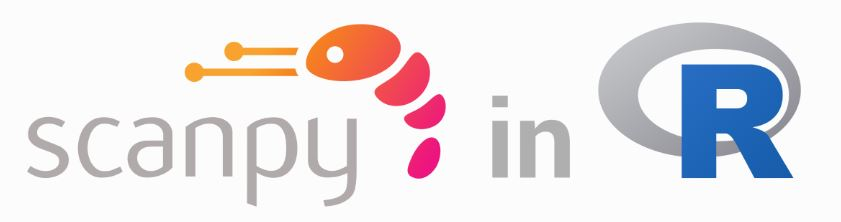#### Introduction

H5ad files were uploaded as available data in some paper of scRNA. But I always analysis scRNA data by seurat package in R. As we known, there is a function named read10X_h5 for reading filtered matrix in seurat package. But seurat package does not include any function to read h5ad file, which were from pipeline of scanpy.So some command is needed to solve this problem.

#### Code exmaple

Installation of reticulate and renv，intall needed package of R and python

``````# Install renv
install.packages("renv")

#We can then ask {renv} to create an environment for us:
renv::init()

#Install {reticulate} package installed in our new renv environment:
renv::install("reticulate")

#We also want to use a Python environment:
renv::use_python()

#install the R packages used during this tutorial:
pkgs <- c(
"renv",
"reticulate",
"png",
"ggplot2",
"BiocManager",
"Seurat"
)

bioc_pkgs <- c(
"SingleCellExperiment",
"scater",
"multtest"
)

# If you are using an {renv} environment
renv::install(pkgs)
# Install Bioconductor packages
BiocManager::install(bioc_pkgs, update = FALSE)
py_pkgs <- c(
"scanpy",
"python-igraph",
"louvain"
)
reticulate::py_install(py_pkgs)

# This records the changes you have made so they can be restored later if necessary
# When you load R in same work path, this enviroment will be restored automatically .
renv::snapshot()
``````

``````library(Seurat)
library(data.table)
library(reticulate)
library(renv)
library(ggplot2)``````

``````sc <- import("scanpy")
setwd("/home/hxzk/project/Qu/Heart/")
``````

``annData = sc\$read_h5ad("data/figshare_8273102/Heart_droplet.h5ad")``

Create seurat object from h5ad data

``````# Create seurat object
exprs <- t(data.matrix(annData\$X))
colnames(exprs) <- annData\$obs_names\$to_list()
rownames(exprs) <- annData\$var_names\$to_list()
# Create the Seurat object
seurat <- CreateSeuratObject(exprs)
# Set the expression assay
seurat <- SetAssayData(seurat, "data", exprs)
# Zscore normalization if we need
norm_expr <- apply(exprs, 2, function(x) (x - mean(x))/sd(x))
seurat[["RNA"]][["n_cells"]] <- annData\$var["n_cells"]
embedding <- annData\$obsm["X_umap"]
rownames(embedding) <- annData\$obs_names\$to_list()
colnames(embedding) <- c("umap_1", "umap_2")
seurat[["umap"]] <- CreateDimReducObject(embedding, key = "umap_")``````

We can plot some figures as we have transformed h5ad file to seurat object successfully

``````
# Check batch effect
pdf("result/figure/batch_age.pdf", width = 4, height = 3.5)
DimPlot(seurat, reduction = "umap",group.by = "batch")
dev.off()

# Show cluster and annotation
pdf("result/figure/cluster.pdf", width = 12, height = 5)
p1 <- DimPlot(seurat, reduction = "umap",group.by = c("louvain"), label = T)
p2 <- DimPlot(seurat, reduction = "umap",group.by = "free_annotation", label = T)
p1 + p2
dev.off()
``````

#### References

https://theislab.github.io/scanpy-in-R/#introduction